GCSE Maths Number FDP Decimals

Here we will learn about adding and subtracting decimals, including calculations with two or more decimals, or with a mixture of decimals and integers.

There are also adding and subtracting decimals worksheets based on Edexcel, AQA and OCR exam questions, along with further guidance on where to go next if you’re still stuck.

## What is adding and subtracting decimals?

Adding and subtracting decimals is the skill of carrying out a calculation involving decimal numbers correctly by understanding place value.

When adding or subtracting with decimals we can use the column method; special care must be taken to ensure that the decimal points line up with each other.

For example, let’s look at 12.5+6.23.

Decimal numbers are used in real life particularly when using measurements such as money, length, mass and capacity. Therefore you may find the skill of adding and subtracting decimals useful when you are problem solving or answering worded questions in context.

On this page we will focus on using the column method to add or subtract terminating positive decimal numbers. No calculations will involve negative numbers or recurring decimals.

For information on calculating with negative numbers and different types of decimal numbers you can follow these links.

Step-by-step guide: Adding and subtracting negative numbers

Step-by-step guide: Recurring decimals

### What is adding and subtracting decimals?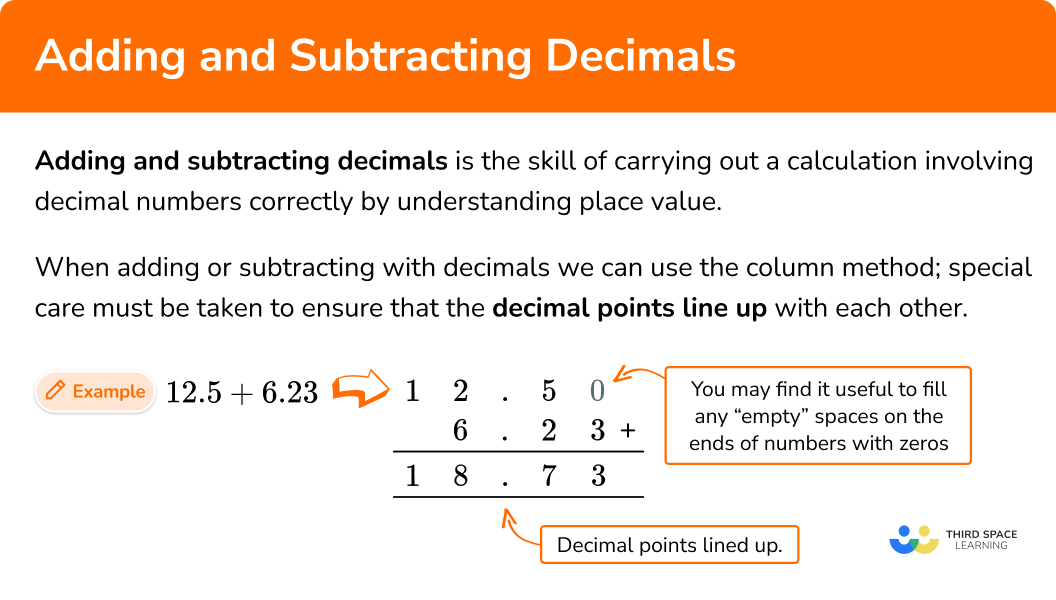## How to add and subtract decimals

In order to add or subtract decimals:

1. Make sure each number has a decimal point and write any \bf{0} placeholders that are required.
2. Write the numbers in a column ensuring that the decimal points line up.
3. Use the column method for addition/subtraction, ensuring the decimal point is also written in the answer.

### Explain how to add and subtract decimals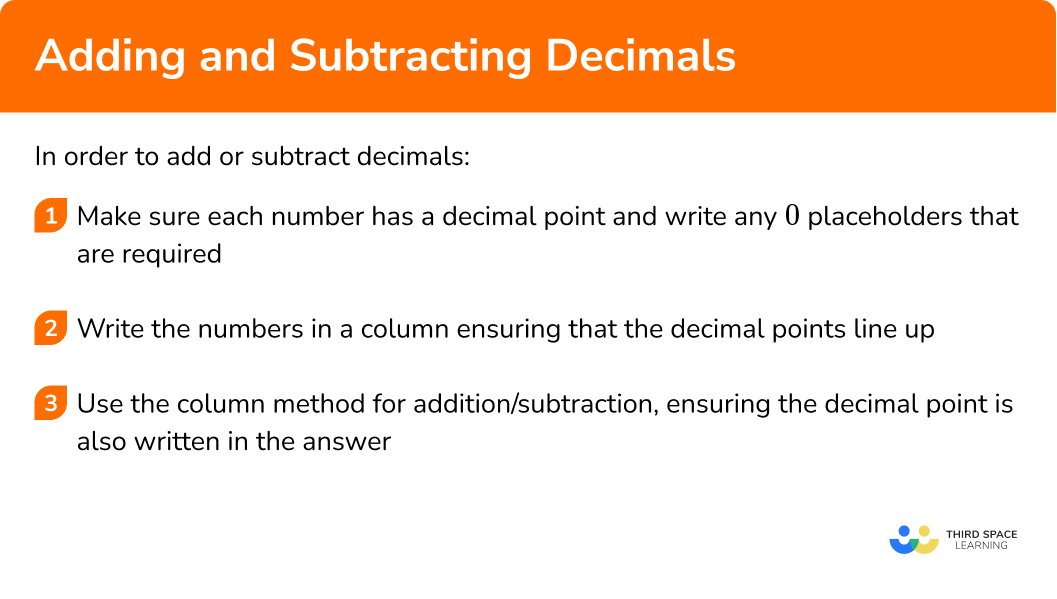### Related lessons on decimals

Adding and subtracting decimals is part of our series of lessons to support revision on decimals. You may find it helpful to start with the main decimals lesson for a summary of what to expect, or use the step by step guides below for further detail on individual topics. Other lessons in this series include:

## Adding and subtracting decimals examples

### Example 1: adding two decimals using column method with no carrying

Calculate 12.3+4.5.

1. Make sure each number has a decimal point and write any \bf{0} placeholders that are required.

Each number has a decimal point and one decimal place so no zero placeholders are required.

2Write the numbers in a column ensuring that the decimal points line up.

3Use the column method for addition/subtraction, ensuring the decimal point is also written in the answer.

Adding the digits in the columns from right to left, we have

Note that the decimal point is placed in the same column in the solution.

So 12.3+4.5=16.8.

### Example 2: adding an integer and a decimal using column method with no carrying

Calculate 52+31.07.

The decimal number contains two decimal places and so we need to write 52 with a decimal point and two 0 placeholders, so we write 52.00.

Adding the digits in the columns from right to left, we have

Note that the decimal point is placed in the same column in the solution.

So 52+31.07=83.07.

### Example 3: adding two decimals using column method with carrying

Calculate 6.7+9.31.

The first number contains one decimal place whereas the second number contains two decimal places and so we need to write a 0 placeholder on the first number. We will therefore write 6.70.

Adding the digits in the columns from right to left, we have

As 7+ 3=10, the 1 digit from the number 10 is placed below the units column, and not below the decimal point.

As 6+9+1 (which we carried over) =16, we need to carry over the new 1 digit to the tens column and write this below the solution line.

So 6.7+9.31=16.01.

### Example 4: subtracting two decimals using column method with no exchanging

Calculate 26.87-14.2.

The two numbers in the question have a different number of decimal places and so we need to write a 0 placeholder on the second number. We will therefore write 14.20.

We subtract the digits in the columns going from right to left ensuring the digit underneath is subtracted from the digit above.

Note that the decimal point is placed in the same column in the solution.

So 26.87-14.2=12.67.

### Example 5: subtracting a decimal from an integer using column method with exchanging

Calculate 16-9.4.

The first number is an integer and the second number contains one decimal place and so we need to write a decimal point and a 0 placeholder on the first number. We will therefore write 16.0.

We subtract the digits in the columns going from right to left ensuring the digit underneath is subtracted from the digit above.

As the digit below is larger than the digit above in the tenths column, we first need to exchange “1” from the units column (leaving us with “5” in this column) for “10” in the tenths column (giving us “10” in this column).

This means that we need to calculate 10-4, which is equal to 6.

Note that the decimal point is placed in the same column in the solution.

As the digit below is larger than the digit above in the units column, we need to use the process of exchanging again.

This time we exchange “1” from the tens column (leaving us with “0” in this column) for “10” in the units column (giving us “15” in this column).

So 16-9.4=6.6.

### Example 6: subtracting two decimals using column method with exchanging

Calculate 2.04-0.952 .

The first number has two decimal places and the second number has three decimal places and so we need to write a 0 placeholder on the first number. We will therefore write 2.040.

We subtract the digits in the columns going from right to left ensuring the digit underneath is subtracted from the digit above.

As the digit below is larger than the digit above in the thousandths column, we first need to exchange “1” from the hundredths column (leaving us with “3” in this column) for “10” in the thousandths column (giving us “10” in this column).

This means that we need to calculate 10-2, which is equal to 8.

As the digit below is larger than the digit above in the hundredths column, we need to use the process of exchanging again.

However, we have an issue because the tenths column contains a 0. This means that we need to do two exchanges.

First we exchange “1” from the units column (leaving us with “1” in this column) for “10” in the tenths column (giving us “10” in this column).

Then we exchange “1” from the tenths column (leaving us with “9” in this column) for “10” in the hundredths column (giving us “13” in this column).

We can now calculate 13-5.

So 2.04-0.952=1.088.

### Common misconceptions

• Lining up decimal numbers in a column incorrectly
When using a column method for adding or subtracting with decimal numbers students can sometimes line up the numbers incorrectly. This is because younger students are sometimes told to line up the numbers from the right hand side, but this method only works for integers.

When lining decimal numbers in a column you must line up the decimal points. This will ensure that the digits are in the correct column according to their place value. Using zero placeholders can also help you to avoid making this mistake.

• Subtracting the smaller digit from the larger digit during a column subtraction even though the smaller digit is above the larger digit
When using a column method for subtracting numbers, students can find themselves automatically subtracting the smaller digit from the larger digit, rather than taking time to note which digit is supposed to be subtracted from the other.

The digit below should always be subtracted from the digit above. If the digit above is smaller than the digit below then a method often referred to as ‘exchanging’ must take place (note that some teachers refer to this method as ‘borrowing’).

For instance, here is the incorrect method for example 5 where the smaller digit which is above has been incorrectly subtracted from the larger digit. Also see the correct method where ‘exchanging’ has taken place.

Incorrect solution:

Correct solution: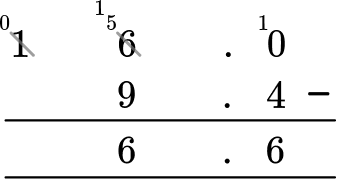• Forgetting to write the decimal point in the solution to a column addition/subtraction involving decimals
When using a column method for addition/subtraction involving decimals, students can sometimes forget to write the decimal point in their solution. It is important to remember to write this, particularly if the answer is not an integer. It is good practice to write the decimal point in the solution area before you start to carry out the calculation. The decimal point should line up with the points above.

Note how the decimal point has been written in the solution area before the calculation has been carried out.

### Practice adding and subtracting decimals questions

1. Calculate 58.1 + 0.46.

62.758.566.275.856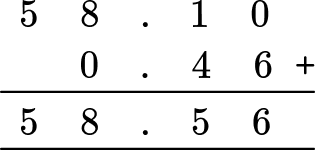2. Calculate 41.3 + 38.

79.341.6841.4145.1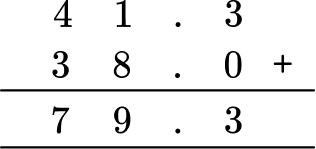3. Calculate 10.62+7.73.

17.2317.13518.3587.92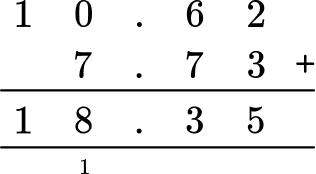4. Calculate 16.9-3.3.

13.616.5713.871.36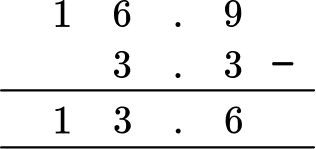5. Calculate 27-1.24.

26.2425.7614.61.46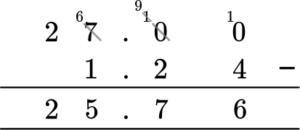6. Calculate 7.11-6.84.

64.261.731.950.27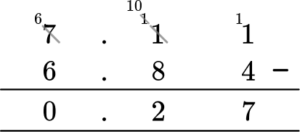### Adding and subtracting decimals GCSE questions

1. This table shows the 4 most recent world records for the men’s 100 metre race.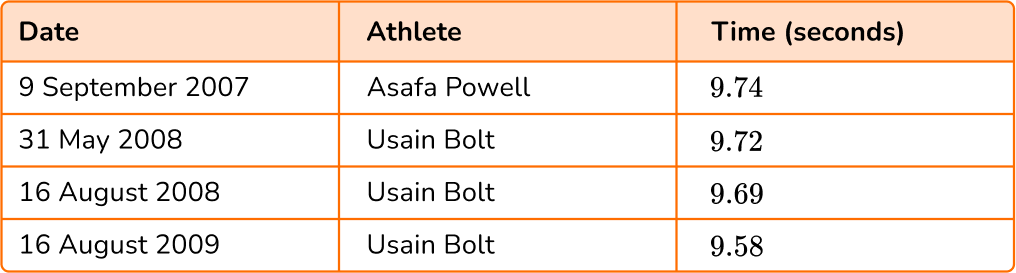Usain Bolt holds the current world record for the men’s 100 metre race at 9.58 seconds. How many seconds did he shave off the previous world record holder’s time?

(1 mark)

9.74-9.58=0.16 seconds

(1)

2. Abi, Bobby and Cyrus each have some money. They want to buy a ball from a local shop costing £3.60  in order to play catch. They decide to put their money together in order to buy the ball.

Abi has £2.30.

Bobby has £1.25.

Cyrus has 9 pence.

If they buy the ball, how much change will they get?

(2 marks)

2.30+1.25+0.09=3.64

3.64-3.60=0.04

(1)

Change is £0.04 or 4 pence.

(1)

3. Ali is harvesting potatoes. He weighs and measures the length of a sample of 10 potatoes. Below is a table showing his results.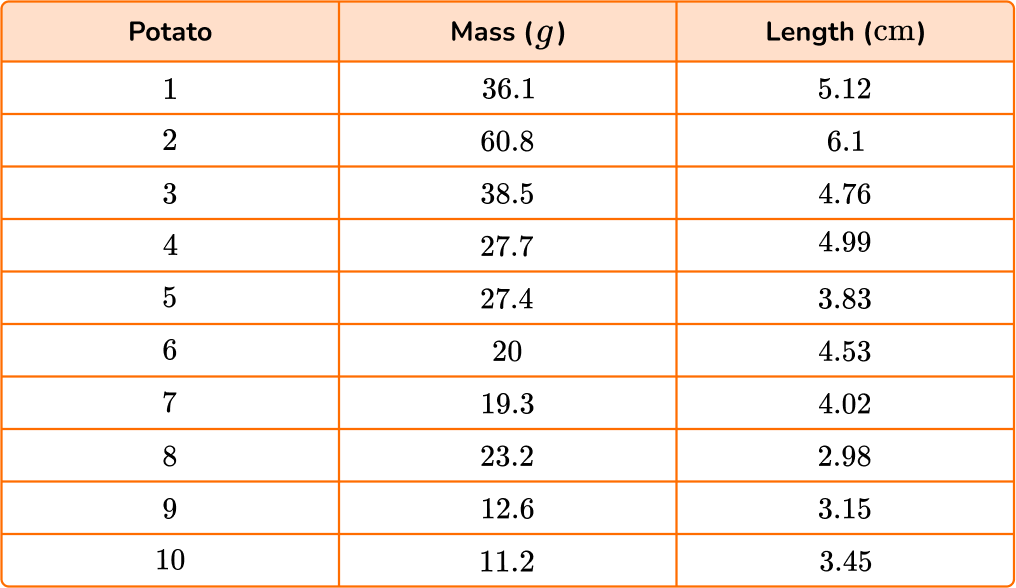(a) Calculate the range for the lengths of the potatoes in the sample.

(b) What is the total mass of the 3 longest potatoes?

(4 marks)

(a) Largest – Smallest =6.1-2.98

(1)

3.12 \, cm

(1)

(b) Potatoes 1, 2, and 4, 36.1 + 60.8 + 27.7

(1)

124.6 \, g

(1)

## Learning checklist

You have now learned how to:

• Use formal written methods for addition and subtraction calculations involving decimals

## Still stuck?

Prepare your KS4 students for maths GCSEs success with Third Space Learning. Weekly online one to one GCSE maths revision lessons delivered by expert maths tutors.

Find out more about our GCSE maths tuition programme.